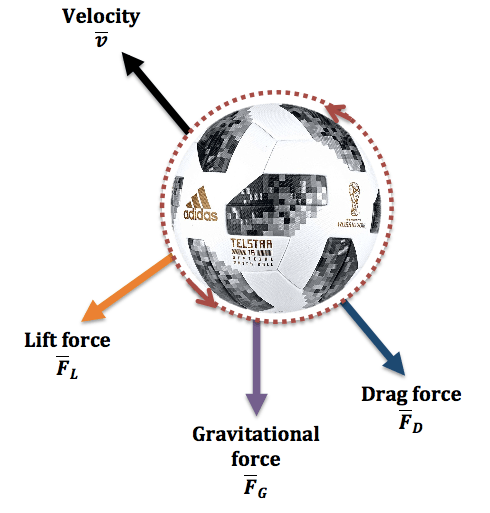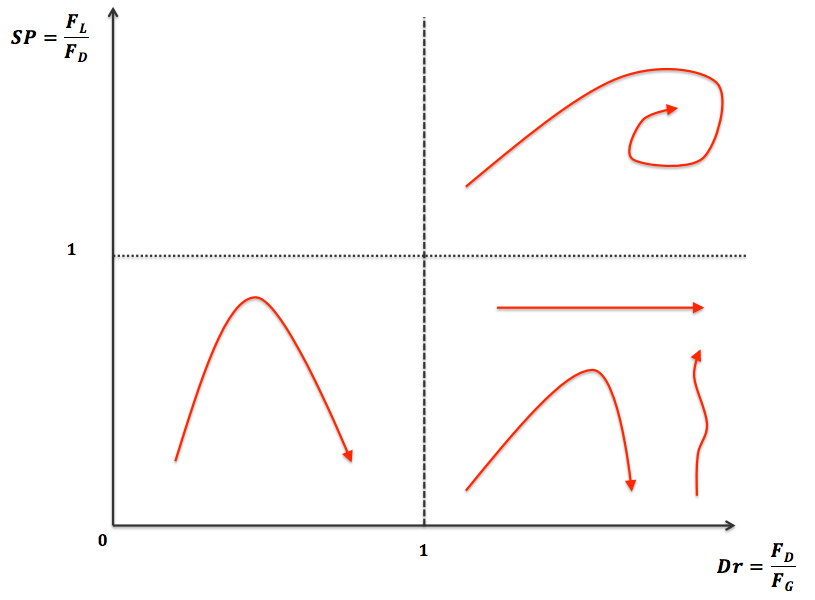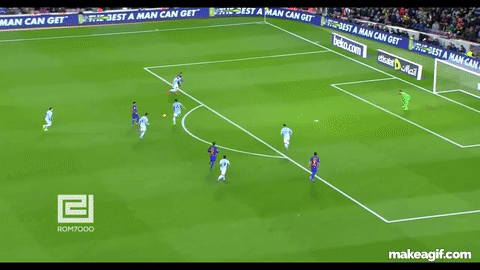# Bend it like Newton: curves in football

Discover the mathematical equations that describe the most commonly observed trajectories in footballBy Agência Brasil - Brasil e Croácia, primeiro jogo da Copa do Mundo de 2014, CC BY 3.0 br, Wikimedia Commons

Football, the most popular sport in the world, has an extensive history. The game we all know in its current form was born in the middle of 19th century. However it has been reported that similar versions existed a long time ago, for instance: ‘the ball game of Mesoamerica’ (2500-100 BCE), Cuju, or Tsu Chu in China (3rd-2nd century BCE), Episkyros in Greece (5th century BCE), to name just a few, which is clear evidence that humankind has enjoyed kicking balls for thousands of years.

In a more recent era, after a meeting in London in 1863 it was established that the ball game would be divided into two main categories: rugby and football association. In addition, one of the main rules for the latter was settled: carrying the ball with one’s hands would not be allowed.

With the passing of time, the game kept evolving and gained popularity among British workers, school teams, etc. Soon, football reached the professional level and the first and oldest official competition, The FA Cup, was created. International matches did not take long to appear, a game between Scotland and England was played in 1872 (which sadly ended up as a boring 0-0 draw).  Then, in the 1920’s, Jules Rimet and a group of French football administrators created the most famous international championship ever, the football World Cup, where the best national football teams from around the world gather every four years to compete for the title of world football champions.

The World Cup begins today in Russia, where 32 teams dream about lifting the most desired trophy. On average, we are expecting to see around 156 goals during the whole competition (which lasts 64 matches). But in order to score a goal, the ball has to be passed between teammates (390 passes per team per game was the average during the last World Cup in Brazil) and it has to be shot on target (27 shots on average per match). In each pass/shot, depending on aerodynamic conditions, we may observe that the ball follows different trajectories: parabolas, straight lines, zig-zags, and many other eye-catching and spectacular curves. Today, we want to explore some of the most commonly observed curves in football, under which conditions it is possible to observe them, and most importantly what are the equations required to describe the trajectory of the ball during each curve.

## Forces affecting a ball’s trajectory

It has been determined that when a ball (with mass $m$) is moving with velocity $U$ through air, there are three main forces that will affect its trajectory: gravitational, drag and lift forces. The diagram below illustrates them.The key forces that determine the trajectory of the official ball that is being used in the current World Cup.

The simplest one is the gravitational force, which is always pointing downwards and is simply defined as $\boldsymbol{F_G}=-m\boldsymbol{g}$, where $\boldsymbol{g}=(0,9.8,0) \mbox{m/s}^2$ is the gravitational acceleration.

#### Aerodynamic forces: drag and lift forces

The other two forces are slightly more complex, and they are always present whenever an object is travelling through a fluid (in our case, a ball flying through air). The first one is the drag force $\boldsymbol{F_D}$, which is the aerodynamic force that opposes a ball’s motion through air. It can be calculated using the following equation:

$\boldsymbol{F_D} = -\frac{1}{2}\rho A C_D|\boldsymbol{v}|\boldsymbol{v},$

where the negative sign means that the force acts in the opposite direction of the ball’s flight. Here, $\rho$ is the air density, $\boldsymbol{v}$ and $|\boldsymbol{v}|$ are the vector velocity and magnitude respectively, $A$ is the cross-sectional area of the football ($A= \pi R^2$, where $R$ is the radius of the ball) and $C_D$ is a parameter that has to be calculated experimentally and tells us how much air resistance will affect the ball’s path. In the absence of air, $C_D=0$.

The last one is called lift or Magnus force $\boldsymbol{F_L}$  and it is useful to explain how spinning balls deviate from typical trajectories that we might expect to see. We previously wrote about the effects of the Magnus force in the world of tennis and football free-kicks, and a detailed explanation is given in both articles. In a few words, when a spinning ball is travelling through air, it drags air faster around one side (upper or bottom side depending if the ball is spinning clockwise or anti-clockwise), which leads to a difference in pressure that creates a sideways force that acts perpendicular to the direction of flight. The equation to calculate the lift force adopts the following form;

$\boldsymbol{F_L} = \frac{1}{2}\rho A C_L|\boldsymbol{v}|\boldsymbol{v} f(\theta),$

where $C_L$ is the lift coefficient (analogous to the drag coefficient for the drag force), which also has to be measured experimentally and depends in many factors such as the angular velocity, linear velocity and diameter of the ball. Lastly, $f(\theta)$ is simply a function that relates the lift force to the angle of spin of the ball.

## Describing a ball’s trajectory: Newton’s second law

In order to describe the path of the football, Newton’s second law is applied. This law simply states that acceleration $\boldsymbol{a}$ of a body (in our case, the ball) will change when it is subjected to external forces (gravitational, drag and lift). Mathematically speaking, the equation is:

$$m \boldsymbol{a} = m \frac{ \mathrm{d}^2 \boldsymbol{x}}{\mathrm{d}t^2} = \boldsymbol{F_G} +\boldsymbol{F_D} + \boldsymbol{F_L},$$

where $\boldsymbol{x}$ is the vector position we are looking for, $\boldsymbol{x}=(x,y,z)$.

## Phase diagram

The solution of the system of second-order differential equations shown above will allow us to describe the path of the ball. As we previously saw, the flight of the ball will depend on the gravitational, lift and drag forces, which also depend on the air density, the initial velocity and the dimensions of the ball, among other parameters.

In many football kicks, one of these forces might dominate over the other two, but there are also cases in which each of them plays an important role. These multiple possibilities cause the variety in the curves that are commonly observed in football, and this motivated a group of French researchers in 2011 to map all of these curves into a simple phase diagram. In this article, we will briefly describe this diagram.

#### Dimensionless numbers: drag and spin numbers

In order to construct the diagram, the research group derived two dimensionless groups. The first one is called the drag number, which is defined as a ratio of the drag and gravitational forces:

$$D_r= \frac{F_D}{F_G},$$

where $F_D$ and $F_G$ are characteristic and scalar values of the vectors $\boldsymbol{F_D}$ and $\boldsymbol{F_G}$, respectively. Assuming that the ball has an initial velocity $U_0$, mass $M$ and radius $R$ and is travelling in $x$-direction, $F_D$ and $F_G$ are:

$$F_D= \frac{1}{2}\rho C_D \pi R^2 U_0^2 \qquad F_G= Mg.$$

It can be easily noticed that if $D_r > 1$, the air resistance dominates over the gravitational effects, and the opposite happens if $D_r < 1$. If $D_r=1$, both forces are equally important.

The other dimensionless number is called the spin number, $SP$, defined as:

$$SP= \frac{F_L}{F_D},$$

where $F_L$ is the scalar value of $\boldsymbol{F_L}$:

$F_L= 2\rho C_L \pi R^3 U_0 \omega_0,$

where $\omega_0$ is the angular velocity that the ball has when it is first hit by the player. Similarly to the drag number, if $SP>1$, the effects of spin on the ball’s trajectory are dominant over air resistance.

#### Phase diagram

Once the dimensionless numbers have been defined, they can be viewed on the following phase diagram:The diagram can be divided in three zones, and below a short explanation is given for each one of them.

#### Gravitational parabola

The first zone occurs when the spin number and the drag force are much smaller than one ($SP < 1$ and $D_r < 1$), which means that the ball will not feel the drag from the surrounding air during its flight and the spin of the ball is not large enough to induce a sideways force perpendicular to the direction of the flight. And additional condition that will cause $SP < 1$ and $D_r <1$ is if the initial velocity of the ball is smaller than some critical number, eg $U_0 \ll 20 \, \mbox{m/s}$). If these conditions are satisfied, Newton’s second law is reduced to:

$$m \frac{ \mathrm{d}^2 \boldsymbol{x}}{\mathrm{d}t^2} = \boldsymbol{F_G} = \, – m \boldsymbol{g},$$

whose solution will give us one of the simplest football trajectories: the gravitational parabola, which is used to describe projectile motions. In football this curve is commonly observed in throw ins, chips (when the ball is hit on the surface and goes over the head of the goalkeeper and lands in the goal), lobs (when the ball is hit on the bounce) and dinks (where the ball has less elevation than the chip).An example of a parabolic trajectory. This chip goal was scored by one of the current World Cup stars: Neymar Jr.

In addition, Newton’s second law for this regime of $D_r <1$ and $SP <1$ is one of the few cases where an analytical solution can be found that describes the ball’s trajectory:

$$y = x \tan \theta \, – \frac{g x^2}{2 U_0^2 \cos ^2 \theta}.$$

Here $y$ and $x$ are the vertical and horizontal displacement of the ball, and $\theta$ is the initial angle of launching of the ball when it was kicked. The solution of the differential equation can also provide us more valuable information, for instance, the maximum horizontal distance $D$ that the ball has travelled when it returns to its original height: $D=(U_0^2/g)\sin (2\theta)$. More equations such as the time of flight ($t=2U_0\sin \theta/g$), the maximum height reached by the ball ($H=U_0^2\sin^2 \theta/2g$), and others can be found here.

#### Straight and zigzag lines

In the previous section we analysed the case where the gravitational force is dominant. Here we will briefly explore what happens when the drag force is dominant, i.e. $SP <1$ and $D_r \gg 1$ and $U_0 >20 \mbox{m/s}$ . In this regime, Newton’s second law is reduced to:

$$m \frac{ \mathrm{d}^2 \boldsymbol{x}}{\mathrm{d}t^2} = \boldsymbol{F_D} = -\frac{1}{2}\rho A C_D|\frac{ \mathrm{d} \boldsymbol{x}}{\mathrm{d}t}| \frac{ \mathrm{d} \boldsymbol{x}}{\mathrm{d}t}.$$

This equation tell us that the velocity of the ball will eventually decrease along the trajectory due to the effects of the drag force. The solution of the differential equation (which can be solved analytically if $C_D$ is constant) gives either straight or zigzag trajectories, depending on the characteristic Reynolds number of the fluid around the ball in flight. Straight trajectories are observed at low Reynolds numbers, and are present in simple passes, simple shots, penalty kicks, etc.

On the other hand, if the drag force increases (and with it the Reynolds number) we have the zigzag trajectory, which is commonly known in football and baseball as the knuckleball effect. All goalkeepers would agree that this curve is a nightmare, as the knuckleball causes an erratic and unpredictable motion that is difficult to stop.

#### Truncated parabola

In the previous cases we observed that if gravity dominates we obtain a simple parabola, and if drag effects play a key role, we can either observe a straight or a zigzag path. But here, we will explain what happens if both forces (gravitational and drag forces) play an equally important role, where Newton’s second law adopts the following form:

$$m \frac{ \mathrm{d}^2 \boldsymbol{x}}{\mathrm{d}t^2} = \boldsymbol{F_G} + \boldsymbol{F_D} =\, -m\boldsymbol{g} -\frac{1}{2}\rho A C_D|\frac{ \mathrm{d} \boldsymbol{x}}{\mathrm{d}t}| \frac{ \mathrm{d} \boldsymbol{x}}{\mathrm{d}t}.$$

The solution of this differential equation is a truncated parabola. The main difference with respect to the gravitational parabola is that after a determined distance $d < D$, (where $D$ was previously defined as the maximum horizontal distance reached by the ball in a gravitational parabola), the ball begins to fall vertically due to high drag effects from the air. The truncated parabola is observed in goal kicks, and explains why in most cases the ball hardly passes beyond the midfield.

#### Spiral

Finally we have the most complex case, in which the spinning effects are highly important, but drag and gravitational forces cannot be neglected because it is known that they play a key role in the ball’s trajectory: $SP \gg 1$ and $D_r > 1$.

For this regime, Newton’s 2nd law adopts the original form given at the beginning of the article, and the solution of the system of differential equations is a spiral trajectory. The effects of a spinning ball have given us spectacular and eye-catching curves, such as the one observed in Roberto Carlos famous free kick in 1997. We previously wrote an special article about it, and a much more detailed analysis with a lot more mathematics is given here.

In this blog we have briefly described some of these curves, but I invite you to read the full article Football curves, where the research group show some of the experiments they conducted in order to prove their observations. In addition, they give a more complete explanation of the (very nice) mathematics behind these trajectories.

Let’s see how many of these football paths we can see during the World Cup!Hugo is a chemical engineer doing a PhD in Mathematics at University College London. He is currently working on non-Newtonian fluid dynamics. He is also interested in transport phenomena and rheology (the science of deformation).
hugocastillocom.wordpress.com    + More articles by Hugo